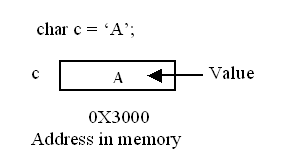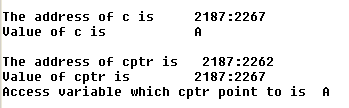# Pointer in C

In the previous tutorial you come to know what is data structure, dynamic memory allocation. Now in this tutorial you will see the how we declare a pointer variable. How it works with a very simple example.

In the previous tutorial you come to know what is data structure, dynamic memory allocation. Now in this tutorial you will see the how we declare a pointer variable. How it works with a very simple example.

A pointer is a variable which contain the memory address. It also points to a specific data types. Three operator are commonly  used  when dealing with pointer.

 1 & address operator 2 * de-referencing operator 3 -> structure pointer operator

Example:

In this example you will see how pointer works.In the above figure you see that c is a variable of char data-type and it has value "A" inside. The address of variable c is 0X3000Now in this figure you see that c is a variable of char data-type and it has value 'A' inside. The address variable c is 0X3000. And a pointer variable cptr of char data-type. The cptr pointer variable having the address of c variable rather value. So this is one the important difference between a general variable and pointer variable is that a pointer variable contains memory address.

## A complete example

#include <stdio.h>
void main()
{
char c ='A';
char *cptr;
cptr=&c;